1593388920

# Time Complexity: How to measure the efficiency of algorithms

In computer programming, as in other aspects of life, there are different ways of solving a problem. These different ways may imply different times, computational power, or any other metric you choose, so we need to compare the efficiency of different approaches to pick up the right one.

Now, as you may know, computers are able to solve problems based on algorithms.

Algorithms are procedures or instructions (set of steps) that tell a computer what to do and how to do it.

Nowadays, they evolved so much that they may be considerably different even when accomplishing the same task. In the most extreme case (which is quite usual by the way), different algorithms programmed in different programming languages may tell different computers with different hardware and operating systems to perform the same task, in a completely different way. That’s crazy, isn’t it?

The thing is that while one algorithm takes seconds to finish, another will take minutes with even small data sets. How can we compare different performances and pick the best algorithm to solve a particular problem?

Fortunately, there are ways of doing this, and we don’t need to wait and see the algorithm at work to know if it can get the job done quickly or if it’s going to collapse under the weight of its input. When we consider the complexity of an algorithm, we shouldn’t really care about the exact number of operations that are performed; instead, we should care about how the number of operations relates to the problem size. Think about it: if the problem size doubles, does the number of operations stay the same? Do they double? Do they increase in some other way? To answer these questions, we need to measure the time complexity of algorithms.

Time complexity represents the number of times a statement is executed. The time complexity of an algorithm is NOT the actual time required to execute a particular code, since that depends on other factors like programming language, operating software, processing power, etc. The idea behind time complexity is that it can measure only the execution time of the algorithm in a way that depends only on the algorithm itself and its input.

To express the time complexity of an algorithm, we use something called the “Big O notation”. **The Big O notation is a language we use to describe the time complexity of an algorithm. **It’s how we compare the efficiency of different approaches to a problem, and helps us to make decisions.

Big O notation expresses the run time of an algorithm in terms of how quickly it grows relative to the input (this input is called “n”). This way, if we say for example that the run time of an algorithm grows “on the order of the size of the input”, we would state that as “O(n)”. If we say that the run time of an algorithm grows “on the order of the square of the size of the input”, we would express it as “O(n²)”. But what does that mean exactly?

The key to understanding time complexity is understanding the rates at which things can grow. The rate in question here is time taken per input size. There are different types of time complexities, so let’s check the most basic ones.

### Constant Time Complexity: O(1)

When time complexity is constant (notated as “O(1)”), the size of the input (n) doesn’t matter. Algorithms with Constant Time Complexity take a constant amount of time to run, independently of the size of n. They don’t change their run-time in response to the input data, which makes them the fastest algorithms out there.

For example, you’d use an algorithm with constant time complexity if you wanted to know if a number is odd or even. No matter if the number is 1 or 9 billions (the input “n”), the algorithm would perform the same operation only once, and bring you the result.

Also, if you wanted to print out once a phrase like the classic “Hello World”, you’d run that too with constant time complexity, since the amount of operations (in this case 1) with this or any other phrase will remain the same, no matter which operating system or which machine configurations you are using.

To remain constant, these algorithms shouldn’t contain loops, recursions or calls to any other non-constant time function. For constant time algorithms, run-time doesn’t increase: the order of magnitude is always 1.

### Linear Time Complexity: O(n)

When time complexity grows in direct proportion to the size of the input, you are facing Linear Time Complexity, or O(n). Algorithms with this time complexity will process the input (n) in “n” number of operations. This means that as the input grows, the algorithm takes proportionally longer to complete.

These are the type of situations where you have to look at every item in a list to accomplish a task (e.g. find the maximum or minimum value). Or you can also think about everyday tasks like reading a book or finding a CD (remember them?) in a CD stack: if all data has to be examined, the larger the input size, the higher the number of operations are.

Linear running time algorithms are very common, and they relate to the fact that the algorithm visits every element from the input.

#2020 jun tutorials #overviews #algorithms #complexity #programming #algorithms

## Buddha Community1593388920

## Time Complexity: How to measure the efficiency of algorithms

In computer programming, as in other aspects of life, there are different ways of solving a problem. These different ways may imply different times, computational power, or any other metric you choose, so we need to compare the efficiency of different approaches to pick up the right one.

Now, as you may know, computers are able to solve problems based on algorithms.

Algorithms are procedures or instructions (set of steps) that tell a computer what to do and how to do it.

Nowadays, they evolved so much that they may be considerably different even when accomplishing the same task. In the most extreme case (which is quite usual by the way), different algorithms programmed in different programming languages may tell different computers with different hardware and operating systems to perform the same task, in a completely different way. That’s crazy, isn’t it?

The thing is that while one algorithm takes seconds to finish, another will take minutes with even small data sets. How can we compare different performances and pick the best algorithm to solve a particular problem?

Fortunately, there are ways of doing this, and we don’t need to wait and see the algorithm at work to know if it can get the job done quickly or if it’s going to collapse under the weight of its input. When we consider the complexity of an algorithm, we shouldn’t really care about the exact number of operations that are performed; instead, we should care about how the number of operations relates to the problem size. Think about it: if the problem size doubles, does the number of operations stay the same? Do they double? Do they increase in some other way? To answer these questions, we need to measure the time complexity of algorithms.

Time complexity represents the number of times a statement is executed. The time complexity of an algorithm is NOT the actual time required to execute a particular code, since that depends on other factors like programming language, operating software, processing power, etc. The idea behind time complexity is that it can measure only the execution time of the algorithm in a way that depends only on the algorithm itself and its input.

To express the time complexity of an algorithm, we use something called the “Big O notation”. **The Big O notation is a language we use to describe the time complexity of an algorithm. **It’s how we compare the efficiency of different approaches to a problem, and helps us to make decisions.

Big O notation expresses the run time of an algorithm in terms of how quickly it grows relative to the input (this input is called “n”). This way, if we say for example that the run time of an algorithm grows “on the order of the size of the input”, we would state that as “O(n)”. If we say that the run time of an algorithm grows “on the order of the square of the size of the input”, we would express it as “O(n²)”. But what does that mean exactly?

The key to understanding time complexity is understanding the rates at which things can grow. The rate in question here is time taken per input size. There are different types of time complexities, so let’s check the most basic ones.

### Constant Time Complexity: O(1)

When time complexity is constant (notated as “O(1)”), the size of the input (n) doesn’t matter. Algorithms with Constant Time Complexity take a constant amount of time to run, independently of the size of n. They don’t change their run-time in response to the input data, which makes them the fastest algorithms out there.

For example, you’d use an algorithm with constant time complexity if you wanted to know if a number is odd or even. No matter if the number is 1 or 9 billions (the input “n”), the algorithm would perform the same operation only once, and bring you the result.

Also, if you wanted to print out once a phrase like the classic “Hello World”, you’d run that too with constant time complexity, since the amount of operations (in this case 1) with this or any other phrase will remain the same, no matter which operating system or which machine configurations you are using.

To remain constant, these algorithms shouldn’t contain loops, recursions or calls to any other non-constant time function. For constant time algorithms, run-time doesn’t increase: the order of magnitude is always 1.

### Linear Time Complexity: O(n)

When time complexity grows in direct proportion to the size of the input, you are facing Linear Time Complexity, or O(n). Algorithms with this time complexity will process the input (n) in “n” number of operations. This means that as the input grows, the algorithm takes proportionally longer to complete.

These are the type of situations where you have to look at every item in a list to accomplish a task (e.g. find the maximum or minimum value). Or you can also think about everyday tasks like reading a book or finding a CD (remember them?) in a CD stack: if all data has to be examined, the larger the input size, the higher the number of operations are.

Linear running time algorithms are very common, and they relate to the fact that the algorithm visits every element from the input.

#2020 jun tutorials #overviews #algorithms #complexity #programming #algorithms1593442500

# Big O Notation and Time/Space Complexity

Big O notation is a commonly used metric used in computer science to classify algorithms based on their time and space complexity. The time and space here is not based on the actual number of operations performed or amount of memory used per se, but rather how the algorithm would scale with an increase or decrease in the amount of data in the input. The notation will represent how an algorithm will run in the worst-case scenario- what is the maximum time or space an algorithm could use? The complexity is written as O(x) where x is the growth rate of the algorithm in regards to n, which is the amount of data input. Throughout the rest of this blog, input will be referred to as n.

# O(1)

O(1) is known as constant complexity. What this implies is that the amount of time or memory does not scale with n at all. For time complexity, this means that n is not iterated on or recursed- generally a value will be selected and returned or a value with be operated on and returned.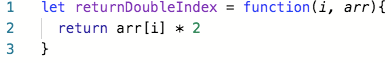Function that returns an index of an array doubled in O(1) time complexity.

For space, no data structures can be created that are a multiples of the size of n. Variables can be declared, but the number must not change with n.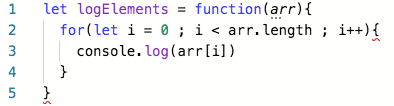Function that logs every element in an array with O(1) space.

# O(logn)

O(logn) is known as logarithmic complexity. The logarithm in O(logn) has a base of 2. The best way to wrap your head around this is to remember the concept of halving: every time n increases by an amount k, the time or space increases by k/2. There are several common algorithms that are O(logn) a vast majority of the time to keep an eye out for: binary search, searching for a term in a binary search tree, and adding items to a heap.

#algorithms #computer-science #big-o-notation #time-complexity #space-complexity #algorithms1624434445

## How to solve time complexity Recurrence Relations using Recursion Tree method?

The Recursion Tree Method is a way of solving recurrence relations. In this method, a recurrence relation is converted into recursive trees. Each node represents the cost incurred at various levels of recursion. To find the total cost, costs of all levels are summed up.

Steps to solve recurrence relation using recursion tree method:

1. Draw a recursive tree for given recurrence relation
2. Calculatethe cost at each level and count the total no of levels in the recursion tree.
3. Count the total number of nodes in the last level and calculate the cost of the last level
4. Sum up the cost of all the levels in the recursive tree

Let us see how to solve these recurrence relations with the help of some examples:

Question 1: T(n) = 2T(n/2) + c

#algorithms #gate cs #recursion #algorithms-analysis of algorithms (recurrences) #time complexity1594945860

## Big O Notation and Time Complexity

Big O notation is a simplified analysis of an algorithm’s efficiency. Big O notation gives us an algorithm’s complexity in terms of input size, N. It gives us a way to abstract the efficiency of our algorithm or code from the machines/computers they run on. We don’t care how powerful our machine is, but rather, the basic steps of the code. We can use big O to analyze both time and space. I will go over how we can use Big O to measure time complexity using Ruby for examples.

Types of measurement

There are a couple of ways to look at an algorithm’s efficiency. We can examine worst-case, best-case, and average-case. When we examine big O notation, we typically look at the worst-case. This isn’t to say the other cases aren’t as important.

General rules

1. Ignore constants

5n ->O(n)

Big O notation ignores constants. For example, if you have a function that has a running time of 5n, we say that this function runs on the order of the big O of N. This is because as N gets large, the 5 no longer matters.

2. In the same way that N grows, certain terms “dominate” others

Here’s a list:

O(1) < O(logn) < O(n) < O(nlogn) < O(n²) < O(2^n) < O(n!)

We ignore low-order terms when they are dominated by high order terms.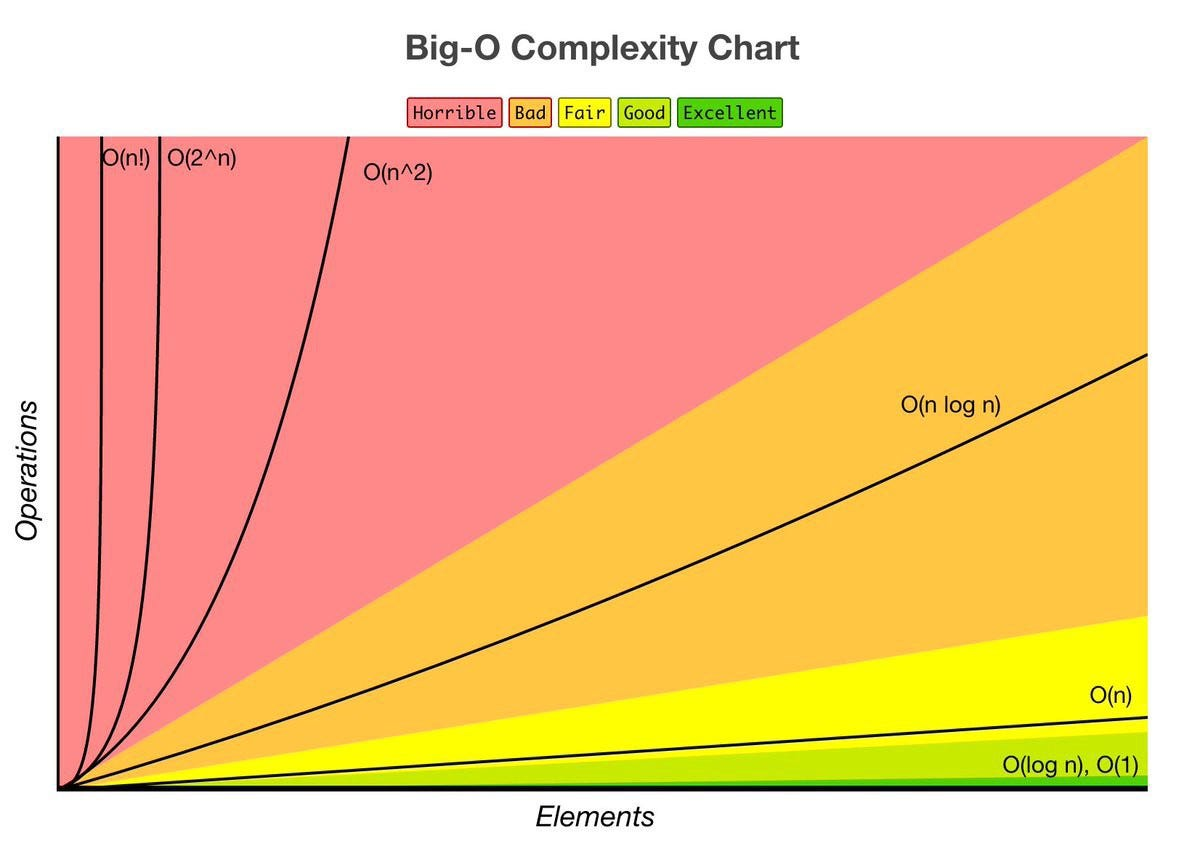Constant Time: O(1)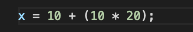This basic statement computes x and does not depend on the input size in any way. This is independent of input size, N. We say this is a “Big O of one” or constant time.

#time-complexity #algorithms #big-o-notation #flatiron-school #algorithms1594945560

## Big O Notation and Time Complexity

Big O notation is a simplified analysis of an algorithm’s efficiency. Big O notation gives us an algorithm’s complexity in terms of input size, N. It gives us a way to abstract the efficiency of our algorithm or code from the machines/computers they run on. We don’t care how powerful our machine is, but rather, the basic steps of the code. We can use big O to analyze both time and space. I will go over how we can use Big O to measure time complexity using Ruby for examples.

Types of measurement

There are a couple of ways to look at an algorithm’s efficiency. We can examine worst-case, best-case, and average-case. When we examine big O notation, we typically look at the worst-case. This isn’t to say the other cases aren’t as important.

General rules

1. Ignore constants

5n ->O(n)

Big O notation ignores constants. For example, if you have a function that has a running time of 5n, we say that this function runs on the order of the big O of N. This is because as N gets large, the 5 no longer matters.

2. In the same way that N grows, certain terms “dominate” others

Here’s a list:

O(1) < O(logn) < O(n) < O(nlogn) < O(n²) < O(2^n) < O(n!)

We ignore low-order terms when they are dominated by high order terms.Constant Time: O(1)This basic statement computes x and does not depend on the input size in any way. This is independent of input size, N. We say this is a “Big O of one” or constant time.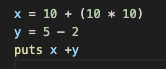total time = O(1) + O(1) + O(1) = O(1)

What happens when we have a sequence of statements? Notice that all of these are constant time. How do we compute big O for this block of code? We simply add each of their times and we get 3 * O(1). But remember we drop constants, so this is still big O of one.

Linear Time: O(n)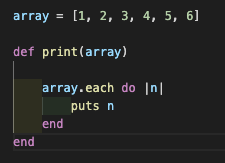The run time of this method is linear or O(n). We have a loop inside of this method which iterates over the array and outputs the element. The number of operations this loop performs will change depending on the size of the array. For example, an array of size 6 will only take 6 iterations, while an array of 18 elements would take 3 times as long. As the input size increases, so will the runtime.Big O notation is a simplified analysis of an algorithm’s efficiency. Big O notation gives us an algorithm’s complexity in terms of input size, N. It gives us a way to abstract the efficiency of our algorithm or code from the machines/computers they run on. We don’t care how powerful our machine is, but rather, the basic steps of the code. We can use big O to analyze both time and space. I will go over how we can use Big O to measure time complexity using Ruby for examples.

Types of measurement

There are a couple of ways to look at an algorithm’s efficiency. We can examine worst-case, best-case, and average-case. When we examine big O notation, we typically look at the worst-case. This isn’t to say the other cases aren’t as important.

General rules

#time-complexity #algorithms #big-o-notation #flatiron-school #algorithms